2021 NBA Most Improved Player of the Year Odds
-285
0.35 to 1Ja Morant
59.6% implied probability

+650
6.5 to 1Miles Bridges
10.7% implied probability

+1000
10 to 1Darius Garland
7.3% implied probability

+1600
16 to 1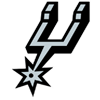Dejounte Murray
4.7% implied probability

+4500
45 to 1Desmond Bane
1.7% implied probability

+5000
50 to 1Jarrett Allen
1.6% implied probability

+8000
80 to 1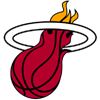Tyler Herro
1.0% implied probability

+8000
80 to 1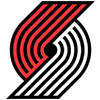Anfernee Simons
1.0% implied probability

+12000
120 to 1Anthony Edwards
0.7% implied probability

+15000
150 to 1Fred VanVleet
0.5% implied probability

+15000
150 to 1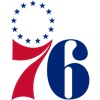Tyrese Maxey
0.5% implied probability

+15000
150 to 1Jordan Poole
0.5% implied probability

+15000
150 to 1LaMelo Ball
0.5% implied probability

+15000
150 to 1Cole Anthony
0.5% implied probability

+20000
200 to 1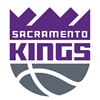Tyrese Haliburton
0.4% implied probability

+20000
200 to 1Jaren Jackson
0.4% implied probability

+20000
200 to 1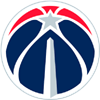Kyle Kuzma
0.4% implied probability

+25000
250 to 1OG Anunoby
0.3% implied probability

+25000
250 to 1Mikal Bridges
0.3% implied probability

+25000
250 to 1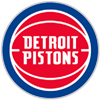Hamidou Diallo
0.3% implied probability

+30000
300 to 1Keldon Johnson
0.3% implied probability

+30000
300 to 1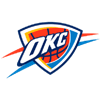Luguentz Dort
0.3% implied probability

+30000
300 to 1Deandre Ayton
0.3% implied probability

+40000
400 to 1Terry Rozier
0.2% implied probability

+40000
400 to 1Robert Williams
0.2% implied probability

+50000
500 to 1Mo Bamba
0.2% implied probability

+50000
500 to 1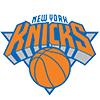RJ Barrett
0.2% implied probability

+50000
500 to 1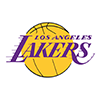Malik Monk
0.2% implied probability

No changes have been recorded yet.
+50000
500 to 1Matisse Thybulle
0.2% implied probability

+50000
500 to 1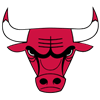Coby White
0.2% implied probability

+50000
500 to 1Saddiq Bey
0.2% implied probability

+50000
500 to 1Terance Mann
0.2% implied probability

+50000
500 to 1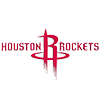Kevin Porter
0.2% implied probability

+50000
500 to 1Cameron Johnson
0.2% implied probability

+50000
500 to 1Daniel Gafford
0.2% implied probability

+50000
500 to 1Immanuel Quickley
0.2% implied probability

+50000
500 to 1P.J. Washington
0.2% implied probability

+50000
500 to 1Nikola Vucevic
0.2% implied probability

+50000
500 to 1Devonte' Graham
0.2% implied probability

+50000
500 to 1Dillon Brooks
0.2% implied probability

+50000
500 to 1Andrew Wiggins
0.2% implied probability

+50000
500 to 1Zach LaVine
0.2% implied probability

+50000
500 to 1D'Angelo Russell
0.2% implied probability

+50000
500 to 1Malik Beasley
0.2% implied probability

+50000
500 to 1Mitchell Robinson
0.2% implied probability

+50000
500 to 1Jaylen Brown
0.2% implied probability

+50000
500 to 1Marvin Bagley
0.2% implied probability

+50000
500 to 1Chris Boucher
0.2% implied probability

+50000
500 to 1Wendell Carter
0.2% implied probability

+50000
500 to 1Derrick White
0.2% implied probability

+50000
500 to 1Seth Curry
0.2% implied probability

+100000
1000 to 1Talen Horton-Tucker
0.1% implied probability

+100000
1000 to 1Jusuf Nurkic
0.1% implied probability

+100000
1000 to 1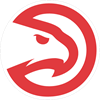Clint Capela
0.1% implied probability

+100000
1000 to 1Alex Caruso
0.1% implied probability

+100000
1000 to 1Bogdan Bogdanovic
0.1% implied probability

+100000
1000 to 1Jordan Clarkson
0.1% implied probability

+100000
1000 to 1Nickeil Alexander-Walker
0.1% implied probability

+100000
1000 to 1Shai Gilgeous-Alexander
0.1% implied probability

+100000
1000 to 1Kristaps Porzingis
0.1% implied probability

+100000
1000 to 1Gary Trent
0.1% implied probability

+100000
1000 to 1Cameron Payne
0.1% implied probability

+100000
1000 to 1Christian Wood
0.1% implied probability

+100000
1000 to 1John Collins
0.1% implied probability

+100000
1000 to 1Bam Adebayo
0.1% implied probability

+100000
1000 to 1Malcolm Brogdon
0.1% implied probability

+100000
1000 to 1Lonzo Ball
0.1% implied probability

+100000
1000 to 1De'Aaron Fox
0.1% implied probability

+100000
1000 to 1Domantas Sabonis
0.1% implied probability